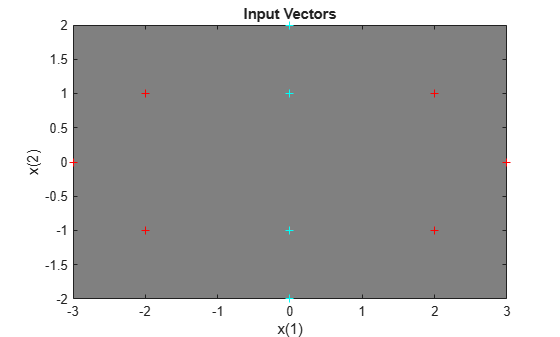# Learning Vector Quantization

An LVQ network is trained to classify input vectors according to given targets.

Let X be 10 2-element example input vectors and C be the classes these vectors fall into. These classes can be transformed into vectors to be used as targets, T, with IND2VEC.

```x = [-3 -2 -2 0 0 0 0 +2 +2 +3; 0 +1 -1 +2 +1 -1 -2 +1 -1 0]; c = [1 1 1 2 2 2 2 1 1 1]; t = ind2vec(c);```

Here the data points are plotted. Red = class 1, Cyan = class 2. The LVQ network represents clusters of vectors with hidden neurons, and groups the clusters with output neurons to form the desired classes.

```colormap(hsv); plotvec(x,c) title('Input Vectors'); xlabel('x(1)'); ylabel('x(2)');```Here LVQNET creates an LVQ layer with four hidden neurons and a learning rate of 0.1. The network is then configured for inputs X and targets T. (Configuration normally an unnecessary step as it is done automatically by TRAIN.)

```net = lvqnet(4,0.1); net = configure(net,x,t);```

The competitive neuron weight vectors are plotted as follows.

```hold on w1 = net.IW{1}; plot(w1(1,1),w1(1,2),'ow') title('Input/Weight Vectors'); xlabel('x(1), w(1)'); ylabel('x(2), w(2)');```To train the network, first override the default number of epochs, and then train the network. When it is finished, replot the input vectors '+' and the competitive neurons' weight vectors 'o'. Red = class 1, Cyan = class 2.

```net.trainParam.epochs=150; net=train(net,x,t); cla; plotvec(x,c); hold on; plotvec(net.IW{1}',vec2ind(net.LW{2}),'o');```Now use the LVQ network as a classifier, where each neuron corresponds to a different category. Present the input vector [0.2; 1]. Red = class 1, Cyan = class 2.

```x1 = [0.2; 1]; y1 = vec2ind(net(x1))```
```y1 = 2 ```